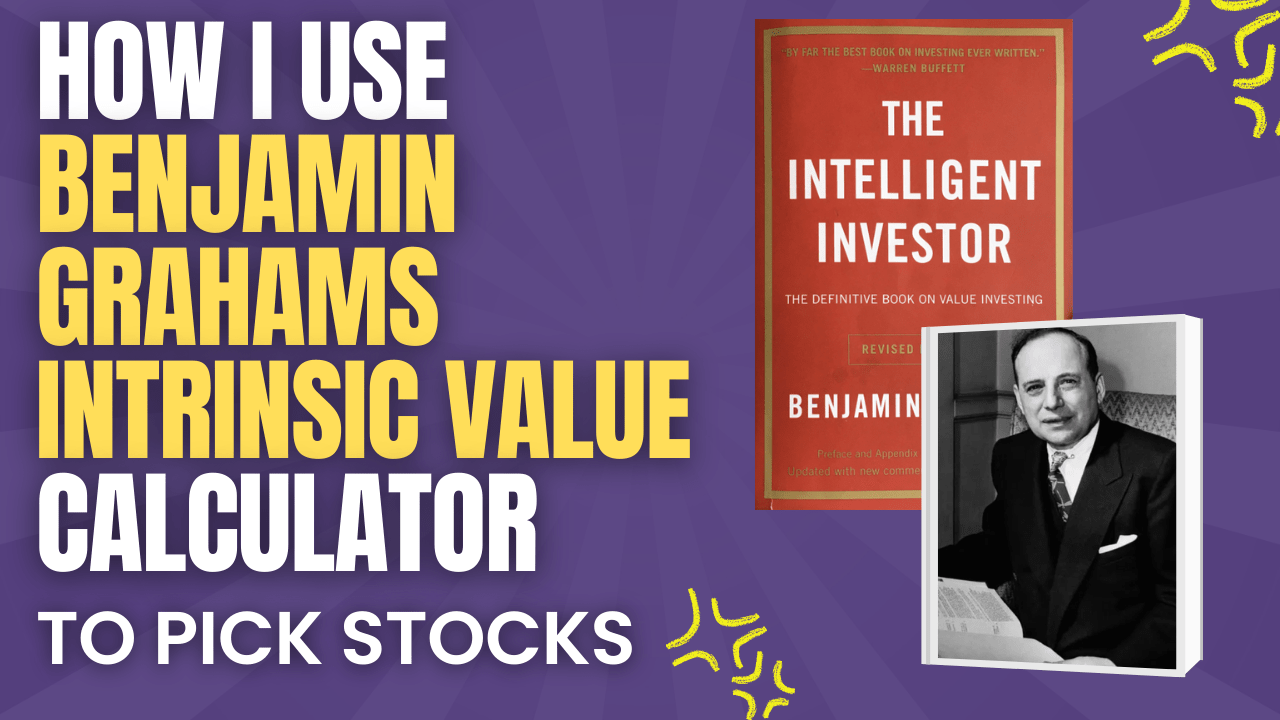# How to Quantify the Leverage (Lambda) of your Options Contract

• By: Walter Dunphy ACCA
• Date: June 29, 2023
• Time to read: 2 min.

One of the benefits of using options (and risks) is that they allow you to multiply your returns be they positive or negative with the use of leverage. When I started trading options I was well aware of this fact, but I couldn’t tell you exactly how much leverage I was getting with each options contract I had.

In this blog post, I will go through a simple way you can calculate the leverage (lambda) of any options contract.

### Options Leverage Calculation

You can calculate the leverage of any options contract by simply multiplying the delta of an option by the price of the underlying stock and then dividing this by the option premium.

λ = Leverage

δ = Delta which is the rate of change in the options premium, due to the change of the price of the underlying stock

### Leverage on an options contract is not fixed

While some types of financial instruments may offer you a fixed amount of leverage, this is not the case with options.

Your leverage will be constantly changing, as the options premium if dependent on many variables such as interest rates, the time to expiry, and the implied volatility.

Generally speaking, leverage is lower for the following

• long dated options (LEAPS) will have a lower leverage, also
• the higher the implied volatility, the lower the leverage

Leverage will be higher in instances where:

• your options contract goes further into the money. This results in the Delta increasing, which then also increases your leverage.

### Real Life Example

Now let us put the formula into practice and work out the leverage on a real life Tesla option.

The contract we are looking at for this example expires on the 4/8/2023 (42 days), with a strike price of \$255. The current share price of Tesla at the time of writing is \$256.96.

The delta of this Tesla option is 0.5698.

When we take all of the above variables into account we come to a leverage of 6.21, which is quite a lot of leverage.

### Refresher on Leverage

If you are need a little refresher on what leverage is, then here is a quick summary.

In options trading, leverage refers to the ability to control a larger position in the market using a smaller amount of capital. Options leverage is achieved through the use of premium payments. When buying an options contract, the trader pays a premium, which is a fraction of the actual cost of owning the underlying asset. This premium allows the trader to control a larger position in the market.

For example, if an options contract gave you leverage at a ratio of 5:1, then if the underlying stock price increased by 1%, then the price of your options contract would increase five fold. Similarly, if the underlying stock price were to fall by 1%, your losses would be magnified by 5.

Disclaimer: this blog post is for informational and educational purposes only and should not be construed as financial advice.Previous Post

Next Post

## Using Benjamin Graham’s Intrinsic Value Calculator to Pick Stocks